|

# 2017全球黑科技大集锦,颠覆三观亮瞎双眼!

2017全球黑科技大集锦，颠覆三观亮瞎双眼！

世界日新月异，黑科技层出不穷，那些曾亮瞎我们双眼的黑科技，有些已成为现实，而有些至今躺在我们的美好幻想中，今天我们聊聊2017年将颠覆人类三观的黑科技。

薄到可以忽略的墙纸电视：LG W7▼

LG 这款电视厚度只有 2.5 毫米，

屏幕和音箱分体式设计，

装在墙上，当窗户使都能以假乱真了，

也被称为“窗户式”OLED 电视。

简直刷新了我对电视的认知。

实在是太太太太薄了，

旁边的老外都目瞪口呆了~

看一眼就亮灯的 LG 冰箱▼

这个冰箱的名字叫 Door in door（门中有门），

敲敲冰箱的面板就亮起来，

经常半夜去冰箱翻东西吃的饿鬼们，

肯定相当喜欢~

冰箱里面还有个广角镜头，

在超市时打开 APP 你就知道缺啥要买的~

冰箱门还支持体感技术，

站到冰箱门前就能自动为你开门，

俩手端盘子也能放东西~

办公室老司机开车必备：本田 Uni-Cub▼

据说本田研发它是为了方便员工在办公室活动的，

倾斜身体就可以前进、后退、横移或旋转，

平时只要把后座上的刹车按下就可以保持稳定，

想移动的时候拉起刹车自己就走了。

只要把重心放在一条腿上暗暗发力就行，

稳定性也是杠杠的，

坐着 Uni-Cub 愉快地奔跑了啊！

另外，本田还展示了一款平衡摩托车！

没有车撑不会倒！这就很牛逼了，

现实款“马良の神笔”：宝丽来 3D 打印笔▼

其实就是把那个类似塑料的材料融化，

然后尽情涂涂画画就行了。

额，不过这个好像老早就有了，

这个是改良版~

小编还上手做过几个手工作品呢~

小孩子也能轻松学会~

电视还能当橱柜玻璃：松下透明电视▼

松下不知道用了什么黑科技，

改变了玻璃的透光度，

让各种看起来普通的玻璃板子都变成了电视，

小编只能说：神奇~

能帮你选下酒菜的智能酒柜▼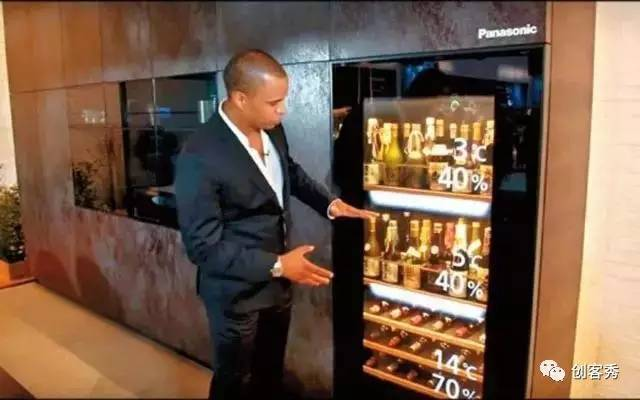这个酒柜可以根据不同酒的种类，

分不同温度/湿度进行保存，

你还可以直接点击柜门查看酒的相关信息，

选择合适的下酒菜，

非常适合酒店装逼式管理。

洗衣烘干巨无霸：三星四合一洗衣机▼这玩意叫 FlexWash + FlexDry 洗衣系统，

看上去就是俩洗衣机，

其实它集四台机器于一体，

俩洗衣机 + 俩烘干机，

全方位解决你的洗衣问题。你可以同时清洗大件和小型衣物，

2台烘干机也是一大一小，

顶部可以清洗/烘干少量易损衣物，

四个部分可以同时工作，也可以独立运转。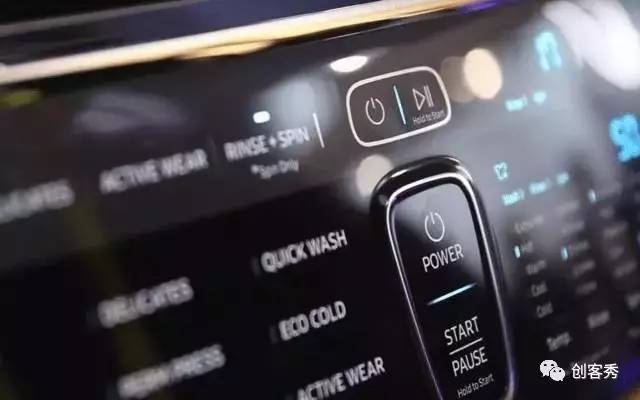黑科技大佬索尼：Bravia OLED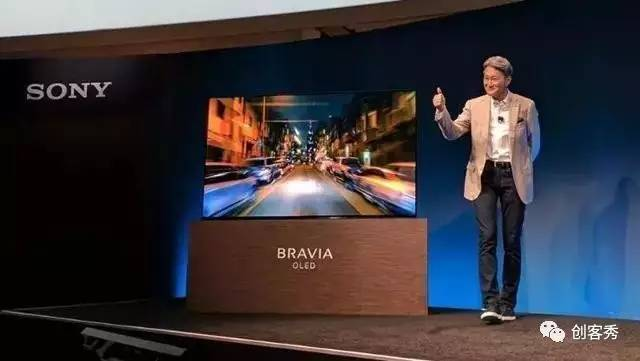四周全窄边框设计，没底座！

那音箱哪去了？别急，

这就是索尼的全新黑科技“平面声场技术”，

可以让屏幕自己震动发声，直接当喇叭使！

索尼果然不吃吹出来的~啥都能控制的小方块：Mesh 智能套件▼索尼还带来了 Mesh 智能家居套件，

简单来说就是一个个可以编程的小方块，

通过App就能给它编程。组合起来可以实现各种功能，

想要什么功能自己动手，

简直不要太棒。唯一的缺点就是一块只能对应一个指令。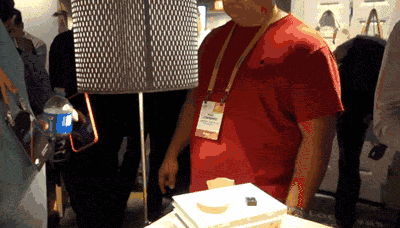三块 4K 屏的游戏神器：雷蛇 Project Valerie▼这台笔记本配备三块 4K 屏幕，

一打开笔记本自动变形三块屏，

让你沉浸在超宽的屏幕里，

（用它打 Dota 会被人说作弊吧）

这要是同时开三个小电影... 那多带劲！

这个相机能拍6K视频：松下 GH5▼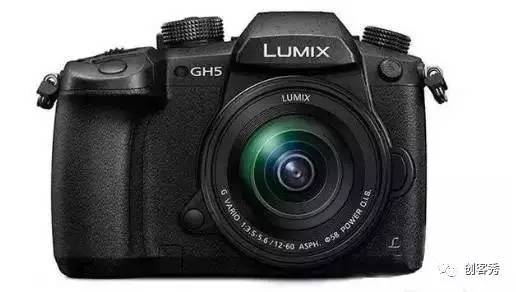这款相机别看器小，但是它活儿好！

4/3小小底面画质奇高，

还能拍6K视频。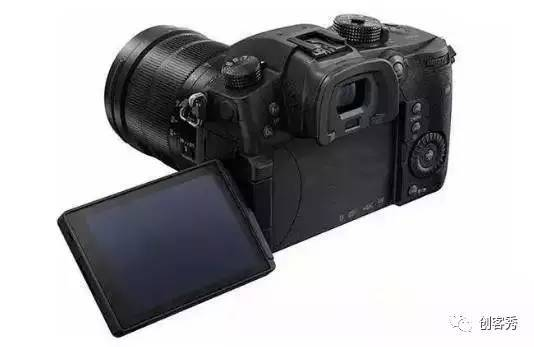表盘表带都能变的智能表：SONY FES Watch▼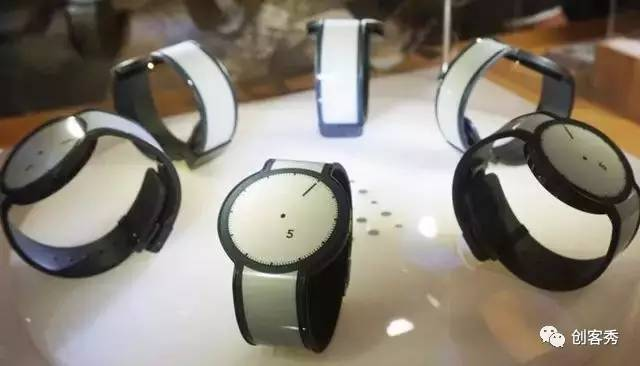索尼这款墨水屏手表，

不光能够变换表盘颜色样式，

甚至连表带都能改变。

彻底解决你戴同一块表审美疲劳的问题。

而且续航超级长，60 天！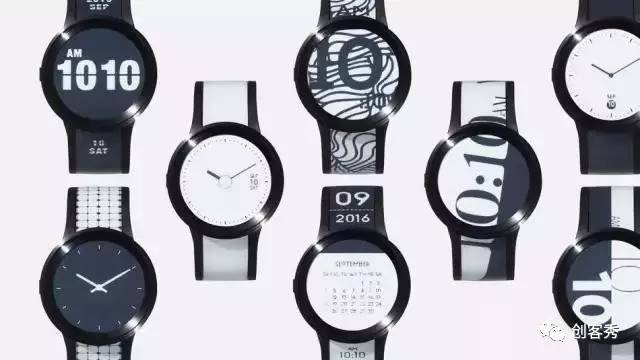再小的房间也能投影：索尼 LSPX-P1 投影仪▼这款索尼便携超短焦投影仪，

只需 28cm 的距离，投出 80 英寸画面，

可以将影像投射在墙上、桌子上，

甚至是小宝宝的画纸上。

不过，5000 多的售价，

小编买不起呀~能检测发质的梳子：Withing Hair Coach欧莱雅竟然和 Withings 的高科技搞到一起了？1

这梳子加上 APP 相当于直接给你一个头发护理师~

梳头的过程中，

可以检测你的发质、头发受损情况和头皮状态，

然后根据你的资料直接推荐给你适合的美发产品，

或者附近的卡诗沙龙，告诉你应该做什么护理，

缺点就是卡诗的洗发水太贵了！懒癌必备：FoldiMate 自动叠衣机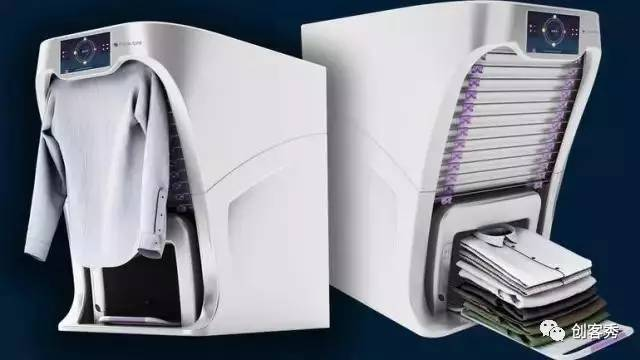懒癌不想叠衣服、手残不会叠的人，

你们的福音来了！

FoldiMate 自动叠衣机，

只要你把衣服放进去 10 秒钟，

就给你吐出来新鲜热乎的衣服，

不过这么小，大件衣服估计没法叠了。

让你身高随意变的高跟鞋▼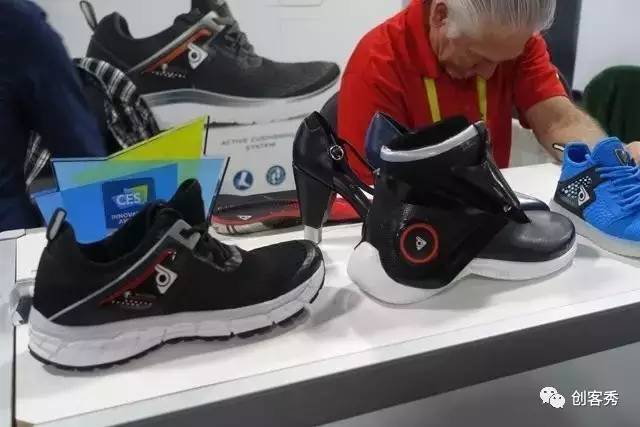姑娘到底买多高的高跟鞋才能对得起自己呢？

一家叫做 ZHOR Tech 的公司成功的吸引了我的注意，

在他们主打产品智能鞋的背后，

藏着一双鞋跟能变长短的高跟鞋，

想要高多少，看你的心情咯~

折纸也能做无人机：Power UP FVP▼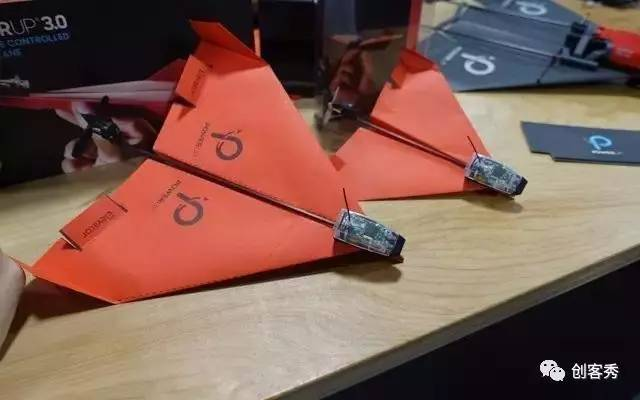专门造纸飞机的 Power UP ，

最近又推出了一款 FVP 飞行器，

只需要在纸飞机上加这个设备，

你就可以通过一个 VR 盒子（也是纸的）来玩 FVP 了，

用第一视角来遥控飞行器，就跟自己坐在上面开飞机一样。智能床：Sleep Number▼Sleep Number 推出的这款 360 智能床，

有一个名叫“睡眠智商”的技术，

躺床上啥也不用戴，

就能监测你的心率、呼吸和运动，

传感器每秒可以检测上百次数据，

来评估你的睡眠质量。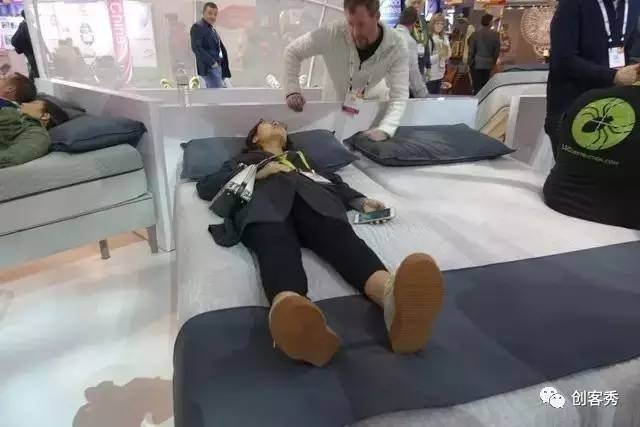床垫还可以调整气囊来适应你的睡姿，

如果翻身了它也跟着调整~

还能调整你的头部姿势，让你停止打鼾！

最让有意思的是它还能暖脚！

根据你的作息时间提前暖好床，

上床就是热乎的！

专治前后上下内外八字脚：ARION 鞋垫▼ATO-GEAR 出了款 ARION 鞋垫，

鞋垫其实是一个多区域的压力传感器，

除了能够检测速度、步频、位置等等之外，

重要的是可以知道你的脚丫子着地时候的受力情况。

帮你改正一些不正确的内外前后上下八字脚，

正确的跑姿会让你跑的更持久，也利于对关节的保护。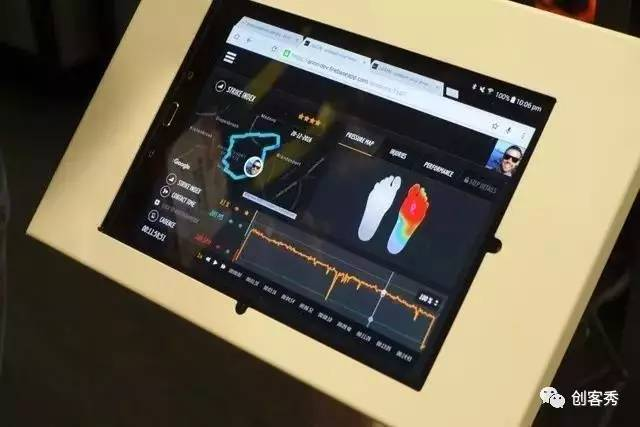会动的“乐高”：优必选 JIMU 机器人▼JIMU 机器人这次加了传感器，

编了一个“来抱抱”的小程序，

只要把手放靠近它就会疯狂赶来拥抱你~

看完这些黑科技，小编有些震撼~

科技好像离我们越来越近，正在无形中彻底颠覆我们的生活~

`声明：本文由入驻焦点开放平台的作者撰写，除焦点官方账号外，观点仅代表作者本人，不代表焦点立场错误信息举报电话： 400-099-0099，邮箱：jubao@vip.sohu.com，或点此进行意见反馈，或点此进行举报投诉。`A B C D E F G H J K L M N P Q R S T W X Y Z
A - B - C - D - E
• A
• 鞍山
• 安庆
• 安阳
• 安顺
• 安康
• 澳门
• B
• 北京
• 保定
• 包头
• 巴彦淖尔
• 本溪
• 蚌埠
• 亳州
• 滨州
• 北海
• 百色
• 巴中
• 毕节
• 保山
• 宝鸡
• 白银
• 巴州
• C
• 承德
• 沧州
• 长治
• 赤峰
• 朝阳
• 长春
• 常州
• 滁州
• 池州
• 长沙
• 常德
• 郴州
• 潮州
• 崇左
• 重庆
• 成都
• 楚雄
• 昌都
• 慈溪
• 常熟
• D
• 大同
• 大连
• 丹东
• 大庆
• 东营
• 德州
• 东莞
• 德阳
• 达州
• 大理
• 德宏
• 定西
• 儋州
• 东平
• E
• 鄂尔多斯
• 鄂州
• 恩施
F - G - H - I - J
• F
• 抚顺
• 阜新
• 阜阳
• 福州
• 抚州
• 佛山
• 防城港
• G
• 赣州
• 广州
• 桂林
• 贵港
• 广元
• 广安
• 贵阳
• 固原
• H
• 邯郸
• 衡水
• 呼和浩特
• 呼伦贝尔
• 葫芦岛
• 哈尔滨
• 黑河
• 淮安
• 杭州
• 湖州
• 合肥
• 淮南
• 淮北
• 黄山
• 菏泽
• 鹤壁
• 黄石
• 黄冈
• 衡阳
• 怀化
• 惠州
• 河源
• 贺州
• 河池
• 海口
• 红河
• 汉中
• 海东
• 怀来
• I
• J
• 晋中
• 锦州
• 吉林
• 鸡西
• 佳木斯
• 嘉兴
• 金华
• 景德镇
• 九江
• 吉安
• 济南
• 济宁
• 焦作
• 荆门
• 荆州
• 江门
• 揭阳
• 金昌
• 酒泉
• 嘉峪关
K - L - M - N - P
• K
• 开封
• 昆明
• 昆山
• L
• 廊坊
• 临汾
• 辽阳
• 连云港
• 丽水
• 六安
• 龙岩
• 莱芜
• 临沂
• 聊城
• 洛阳
• 漯河
• 娄底
• 柳州
• 来宾
• 泸州
• 乐山
• 六盘水
• 丽江
• 临沧
• 拉萨
• 林芝
• 兰州
• 陇南
• M
• 牡丹江
• 马鞍山
• 茂名
• 梅州
• 绵阳
• 眉山
• N
• 南京
• 南通
• 宁波
• 南平
• 宁德
• 南昌
• 南阳
• 南宁
• 内江
• 南充
• P
• 盘锦
• 莆田
• 平顶山
• 濮阳
• 攀枝花
• 普洱
• 平凉
Q - R - S - T - W
• Q
• 秦皇岛
• 齐齐哈尔
• 衢州
• 泉州
• 青岛
• 清远
• 钦州
• 黔南
• 曲靖
• 庆阳
• R
• 日照
• 日喀则
• S
• 石家庄
• 沈阳
• 双鸭山
• 绥化
• 上海
• 苏州
• 宿迁
• 绍兴
• 宿州
• 三明
• 上饶
• 三门峡
• 商丘
• 十堰
• 随州
• 邵阳
• 韶关
• 深圳
• 汕头
• 汕尾
• 三亚
• 三沙
• 遂宁
• 山南
• 商洛
• 石嘴山
• T
• 天津
• 唐山
• 太原
• 通辽
• 铁岭
• 泰州
• 台州
• 铜陵
• 泰安
• 铜仁
• 铜川
• 天水
• 天门
• W
• 乌海
• 乌兰察布
• 无锡
• 温州
• 芜湖
• 潍坊
• 威海
• 武汉
• 梧州
• 渭南
• 武威
• 吴忠
• 乌鲁木齐
X - Y - Z
• X
• 邢台
• 徐州
• 宣城
• 厦门
• 新乡
• 许昌
• 信阳
• 襄阳
• 孝感
• 咸宁
• 湘潭
• 湘西
• 西双版纳
• 西安
• 咸阳
• 西宁
• 仙桃
• 西昌
• Y
• 运城
• 营口
• 盐城
• 扬州
• 鹰潭
• 宜春
• 烟台
• 宜昌
• 岳阳
• 益阳
• 永州
• 阳江
• 云浮
• 玉林
• 宜宾
• 雅安
• 玉溪
• 延安
• 榆林
• 银川
• Z
• 张家口
• 镇江
• 舟山
• 漳州
• 淄博
• 枣庄
• 郑州
• 周口
• 驻马店
• 株洲
• 张家界
• 珠海
• 湛江
• 肇庆
• 中山
• 自贡
• 资阳
• 遵义
• 昭通
• 张掖
• 中卫

1室1厅1厨1卫1阳台

1
2
3
4
5

0
1
2

1

1

0
1
2
3报名成功，资料已提交审核A B C D E F G H J K L M N P Q R S T W X Y Z
A - B - C - D - E
• A
• 鞍山
• 安庆
• 安阳
• 安顺
• 安康
• 澳门
• B
• 北京
• 保定
• 包头
• 巴彦淖尔
• 本溪
• 蚌埠
• 亳州
• 滨州
• 北海
• 百色
• 巴中
• 毕节
• 保山
• 宝鸡
• 白银
• 巴州
• C
• 承德
• 沧州
• 长治
• 赤峰
• 朝阳
• 长春
• 常州
• 滁州
• 池州
• 长沙
• 常德
• 郴州
• 潮州
• 崇左
• 重庆
• 成都
• 楚雄
• 昌都
• 慈溪
• 常熟
• D
• 大同
• 大连
• 丹东
• 大庆
• 东营
• 德州
• 东莞
• 德阳
• 达州
• 大理
• 德宏
• 定西
• 儋州
• 东平
• E
• 鄂尔多斯
• 鄂州
• 恩施
F - G - H - I - J
• F
• 抚顺
• 阜新
• 阜阳
• 福州
• 抚州
• 佛山
• 防城港
• G
• 赣州
• 广州
• 桂林
• 贵港
• 广元
• 广安
• 贵阳
• 固原
• H
• 邯郸
• 衡水
• 呼和浩特
• 呼伦贝尔
• 葫芦岛
• 哈尔滨
• 黑河
• 淮安
• 杭州
• 湖州
• 合肥
• 淮南
• 淮北
• 黄山
• 菏泽
• 鹤壁
• 黄石
• 黄冈
• 衡阳
• 怀化
• 惠州
• 河源
• 贺州
• 河池
• 海口
• 红河
• 汉中
• 海东
• 怀来
• I
• J
• 晋中
• 锦州
• 吉林
• 鸡西
• 佳木斯
• 嘉兴
• 金华
• 景德镇
• 九江
• 吉安
• 济南
• 济宁
• 焦作
• 荆门
• 荆州
• 江门
• 揭阳
• 金昌
• 酒泉
• 嘉峪关
K - L - M - N - P
• K
• 开封
• 昆明
• 昆山
• L
• 廊坊
• 临汾
• 辽阳
• 连云港
• 丽水
• 六安
• 龙岩
• 莱芜
• 临沂
• 聊城
• 洛阳
• 漯河
• 娄底
• 柳州
• 来宾
• 泸州
• 乐山
• 六盘水
• 丽江
• 临沧
• 拉萨
• 林芝
• 兰州
• 陇南
• M
• 牡丹江
• 马鞍山
• 茂名
• 梅州
• 绵阳
• 眉山
• N
• 南京
• 南通
• 宁波
• 南平
• 宁德
• 南昌
• 南阳
• 南宁
• 内江
• 南充
• P
• 盘锦
• 莆田
• 平顶山
• 濮阳
• 攀枝花
• 普洱
• 平凉
Q - R - S - T - W
• Q
• 秦皇岛
• 齐齐哈尔
• 衢州
• 泉州
• 青岛
• 清远
• 钦州
• 黔南
• 曲靖
• 庆阳
• R
• 日照
• 日喀则
• S
• 石家庄
• 沈阳
• 双鸭山
• 绥化
• 上海
• 苏州
• 宿迁
• 绍兴
• 宿州
• 三明
• 上饶
• 三门峡
• 商丘
• 十堰
• 随州
• 邵阳
• 韶关
• 深圳
• 汕头
• 汕尾
• 三亚
• 三沙
• 遂宁
• 山南
• 商洛
• 石嘴山
• T
• 天津
• 唐山
• 太原
• 通辽
• 铁岭
• 泰州
• 台州
• 铜陵
• 泰安
• 铜仁
• 铜川
• 天水
• 天门
• W
• 乌海
• 乌兰察布
• 无锡
• 温州
• 芜湖
• 潍坊
• 威海
• 武汉
• 梧州
• 渭南
• 武威
• 吴忠
• 乌鲁木齐
X - Y - Z
• X
• 邢台
• 徐州
• 宣城
• 厦门
• 新乡
• 许昌
• 信阳
• 襄阳
• 孝感
• 咸宁
• 湘潭
• 湘西
• 西双版纳
• 西安
• 咸阳
• 西宁
• 仙桃
• 西昌
• Y
• 运城
• 营口
• 盐城
• 扬州
• 鹰潭
• 宜春
• 烟台
• 宜昌
• 岳阳
• 益阳
• 永州
• 阳江
• 云浮
• 玉林
• 宜宾
• 雅安
• 玉溪
• 延安
• 榆林
• 银川
• Z
• 张家口
• 镇江
• 舟山
• 漳州
• 淄博
• 枣庄
• 郑州
• 周口
• 驻马店
• 株洲
• 张家界
• 珠海
• 湛江
• 肇庆
• 中山
• 自贡
• 资阳
• 遵义
• 昭通
• 张掖
• 中卫• 手机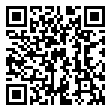• 分享
• 设计
免费设计
• 计算器
装修计算器
• 入驻
合作入驻
• 联系
联系我们
• 置顶
返回顶部